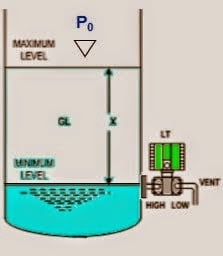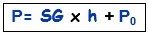Home » Open Tank DP Level Transmitter Calibration

Open Tank DP Level Transmitter Calibration

Schematic Diagram of a open tank DP level transmitter installationOpen Tank DP Level Transmitter CalibrationFormulas:

Min range = SG x h + P0
Max range = SG x h + P 0

Example:

If an X height is 10 meters and there is fluid in the tank which has a density of 0.85 g / cm3 what the calibration range of the transmitter?

SG = 0.85

Min range = SG x h + P0

= 0.85 x 0 m + 0
= 0 mH 2 O

Max range = SG x h + P 0

= 0.85 x 10 m + 0
= 8.5 mH 2 O

The transmitter calibration Range = 0 to 8.5 mH 2 O

Bubble Tube Level Measurement PrincipleFebruary 28, 2017 at 3:43 pm

good evening sir,
what is SG,P0 plz tell meMay 9, 2018 at 8:08 pm

Sir What is P0May 10, 2018 at 2:08 pm

Hi,

SG is the specific gravity of the liquid inside the tank, P0 is the pressure of vapor inside the Tank , if the Tank is open to atmosphere than P0 = 1 bara = 0 barg. In the case above you can see that he is using P0 = 0 barg in the calculation because it is said that in this situation it is an open tank.

This website uses cookies to improve your experience. We'll assume you're ok with this, but you can opt-out if you wish. Accept Read More

WordPress Image Lightbox

Send this to a friend